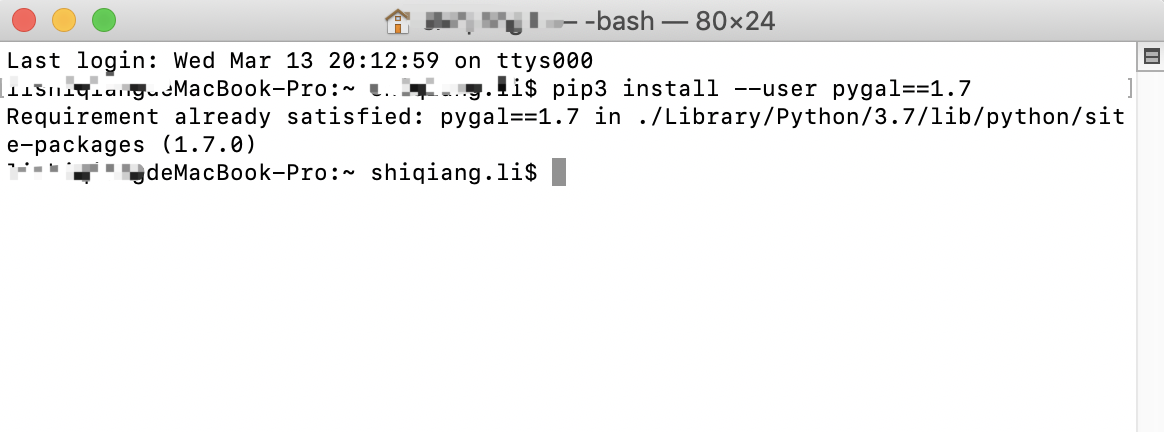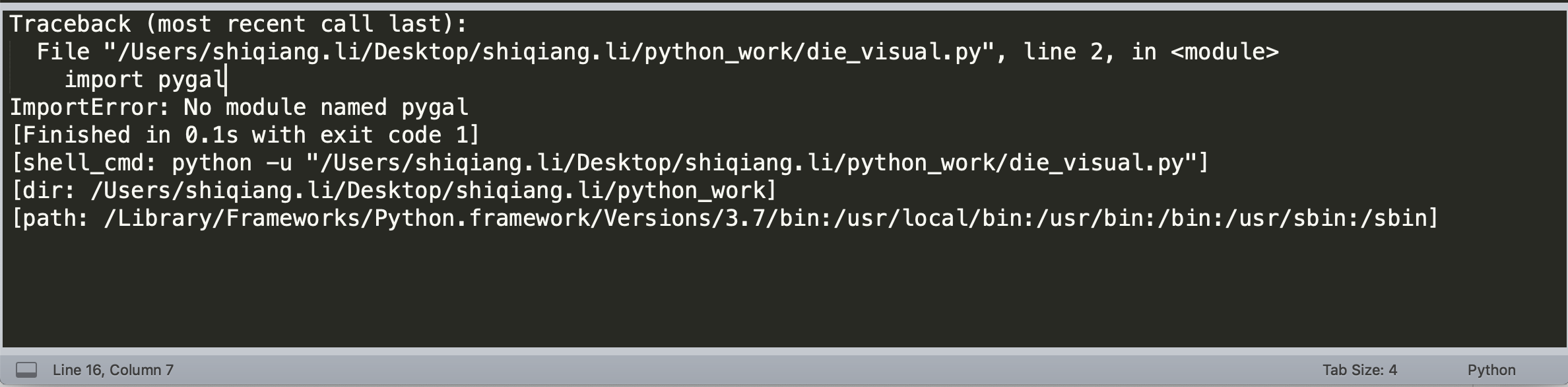python无法倒入pygal，报错ImportError: No module named pygal怎么解决

python版本3.7，确保已使用pip3正确安装pygal。但是无法导入，编译器sublime，卡了很久了，求大佬们帮帮忙！python小白感激不尽！`````` #coding:utf-8
import pygal
from die import Die

# 创建一个D6
die = Die()

# 掷几次骰子，并将结果存储在一个列表中
results = []

# 掷骰子1000次
for roll_num in range(1000):
result = die.roll()
results.append(result)

# 分析结果
frequencies = []
for value in range(1, die.num_sides+1):
frequency = results.count(value)
frequencies.append(frequency)

# 对结果进行可视化
# pygal.Bar() 实例 用于创建条形图，存储到hist中
hist = pygal.Bar()

hist.title = 'Results of rolling one D6 1000 times.'
hist.x_labels = ['1', '2', '3', '4', '5', '6']
hist.x_title = 'Result'
hist.y_title = 'Frequency of Result'

hist.render_to_file('die_visual.svg')
``````

3个回答

1、你如果联网了，在CMD窗口安装pypal库
pip install pypal
2、如果没联网，你先在能联网的机子上去下载,https://www.lfd.uci.edu/~gohlke/pythonlibs/http://pygal.org去，

pip install ‘下载的文件’

1.请确定有没有安装python虚拟环境
2.装有pygal包的python 与 运行 py文件的python是不是同一个
3.py文件所在的文件夹，有没有 pygal 同名文件夹slevenxulianjie 不是一定要安装虚拟环境，是怕你做了虚拟环境，多个python混乱了
11 个月之前 回复11 个月之前 回复python使用pygal报错，麻烦大家看看，帮帮忙！谢谢！
python使用pygal报错：
ValueError: Invalid PI name 'b'xml'' 相关代码：hist.render_to_file('1.svg') 详细错误： ![图片说明](https://img-ask.csdn.net/upload/201708/05/1501922019_825617.png) I have try to solve it by install new version of lxml,but can't work just like this blog.csdn.net/shuxue051/article/details/47686761 I hope somenoe can help me to solve it,Thank you!

ModuleNotFoundError: No module named 'pygal.i18n'
python 里面pygal 。。。。。。。。。
# 我使用pip导入了pygal1.7.为什么提示没有这个模块
python中绘制世界地图TypeError: argument of type 'Key' is not iterable。

ython.pygal/reuqests学习范例-爬取github上Python热度

python从入门到实践，制作交易收盘价走势图

<python从入门到实践>中16章绘制周日均值问题，救救我
``` import json import pygal import math from itertools import groupby filename = 'btc_close_2017.json' with open(filename) as f: btc_data = json.load(f) dates = [] months = [] weeks = [] weekdays = [] close = [] for btc_dict in btc_data: dates.append(btc_dict['date']) months.append((btc_dict['month'])) weeks.append(btc_dict['week']) weekdays.append(btc_dict['weekday']) close.append(int(float(btc_dict['close']))) def draw_line(x_data,y_data,title,y_legend): xy_map = [] for x,y in groupby(sorted(zip(x_data,y_data)),key = lambda _: _): y_list = [v for _,v in y] xy_map.append([x,sum(y_list)/len(y_list)]) x_unique,y_mean = [*zip(*xy_map)] line_chart = pygal.Line() line_chart.title = title line_chart.x_labels = x_unique line_chart.add(y_legend,y_mean) line_chart.render_to_file(title + '.svg') return line_chart idx_week = dates.index('2017-12-11') line_chart_week = draw_line(weeks[1:idx_week],close[1:idx_week],'收盘价周日均值','周日均只') line_chart_week ```
Python制作交易收盘价走势图中天均值，语句wd.index(w) + 1 for w in weekdays[1:idx_week]是什么意思
import json filename = 'btc_close_2017.json' with open(filename) as f: btc_data = json.load(f) dates, months, weeks, weekdays, closes = [], [], [], [], [] for btc_dict in btc_data: dates.append(btc_dict['date']) months.append(int(btc_dict['month'])) weeks.append(int(btc_dict['week'])) weekdays.append(btc_dict['weekday']) closes.append(int(float(btc_dict['close']))) import pygal import math from itertools import groupby def draw_line(x_data, y_data, title, y_legend): xy_map = [] for x, y in groupby(sorted(zip(x_data, y_data)), key=lambda _ : _): y_list = [v for _, v in y] xy_map.append([x, sum(y_list) / len(y_list)]) x_unique, y_mean = [*zip(*xy_map)] line_chart = pygal.Line() line_chart.title = title line_chart.x_labels = x_unique line_chart.add(y_legend, y_mean) line_chart.render_to_file(title+'.svg') return line_chart def draw_line_weekday(x_data, y_data, title, y_legend): xy_map = [] for x, y in groupby(sorted(zip(x_data, y_data)), key=lambda _ : _): y_list = [v for _, v in y] xy_map.append([x, sum(y_list) / len(y_list)]) x_unique, y_mean = [*zip(*xy_map)] line_chart = pygal.Line() line_chart.title = title #line_chart.x_labels = x_unique line_chart.x_labels = ['周一','周二','周三','周四','周五','周六','周日'] line_chart.add(y_legend, y_mean) line_chart.render_to_file(title+'.svg') return line_chart idx_week = dates.index('2017-12-11') wd = ['Monday', 'Tuesday','Wednesday','Thursday','Friday','Saturday','Sunday'] weekdays_int = [wd.index(w) + 1 for w in weekdays[1:idx_week]] line_chart_weekday = draw_line_weekday(weekdays_int, closes[1:idx_week], '收盘价周均值(￥)', '周均值') line_chart_weekday

from itertools import groupby import pygal import json #将数据加载到一个列表中 filename = 'btc_close_2017.json' with open(filename) as f: btc_data = json.load(f) dates = [] months = [] weeks = [] weekdays = [] close = [] #每一天的信息 for btc_dict in btc_data: dates.append(btc_dict['date']) months.append(int(btc_dict['month'])) weeks.append(int(btc_dict['week'])) weekdays.append(btc_dict['weekday']) close.append(int(float(btc_dict['close']))) def draw_line(x_data, y_data, title, y_legend): xy_map = [] for x,y in groupby(sorted(zip(x_data, y_data)), key=lambda _: _): y_list = [v for _, v in y] xy_map.append([x, sum(y_list)/len(y_list)]) x_unique, y_mean = [*zip(*xy_map)] line_chart = pygal.Line() line_chart.title = title line_chart.x_labels = x_unique line_chart.add(y_legend, y_mean) line_chart.render_to_file(title + '.svg') return line_chart idx_month = dates.index('2017-12-01') line_chart_month = draw_line(months[:idx_month], close[:idx_month], '收盘价月日均值', '月日均值') line_chart_month

![图片说明](https://img-ask.csdn.net/upload/201910/29/1572324251_284504.png) ``` import json import pygal import math from itertools import groupby #将数据加载到一个列表中 filename = 'btc_close_2017.json' with open(filename) as f: btc_date = json.load(f) #创建5个列表，分别存储日期和收盘价 dates,months,weeks,weekdays,close = [],[],[],[],[] #打印每一天的信息 for btc_dict in btc_date: dates.append(btc_dict['date']) months.append(btc_dict['month']) weeks.append(btc_dict['week']) weekdays.append(btc_dict['weekday']) close.append(float(btc_dict['close'])) #绘制收盘价折线图 line_chart = pygal.Line(x_label_rotation=20, show_minor_x_labels=False) line_chart.title = '收盘价对数变换' line_chart.x_labels = dates N = 20 #x轴坐标每隔20 显示 line_chart.x_labels_major = dates[::N] close_log = [math.log10(_) for _ in close] line_chart.add('log收盘价',close_log) line_chart.render_to_file('收盘价对数变换折线图.svg') def draw_line(x_data, y_data, title, y_legend): xy_map = [] for x, y in groupby(sorted(zip(x_data, y_data)), key=lambda _: _): y_list = [v for _, v in y] xy_map.append([x, sum(y_list) / len(y_list)]) x_unique, y_mean = [*zip(*xy_map)] line_chart = pygal.Line() line_chart.title = title line_chart.x_labels = x_unique line_chart.add(y_legend, y_mean) line_chart.render_to_file(title+ '.svg') return line_chart idx_month = dates.index('2017-12-01') line_chart_month = draw_line(months[:idx_month], close[:idx_month], '收盘价月日均值', '月日均值') line_chart_month idx_week = dates.index('2017-12-11') line_chart_week = draw_line(weeks[1:idx_week], close[1:idx_week], '收盘价周日均值', '周日均值') line_chart_week ```

python小白一枚，我在用pygal生成的几个svg图片，想把他们放到一个 html文件里面，但是生成的html文件打开是空白。 这个代码在《python编程从入门到实践》16章里面有。的谁能帮我解决问题？ 代码如下： #书本里没有import package ``` with open("收盘价Dashboard.html",'a',encoding='utf-8') as html_file: html_file.write('<html><head><title>收盘价Dashboard</title><meta charset="utf-8"></head><body>\n') for svg in ['收盘折线图.svg','收盘价对数变换折线图.svg', '收盘价月日均收益￥.svg', '收盘价周日均值￥.svg',"收盘价星期均值￥.svg"]: html_file.write(' <object type="image/svg+xml" date="{0}"' 'height=500></object>\n'.format(svg)) html_file.write('</body></html>') ```

python小白一枚，我在用pygal生成的几个svg图片，想把他们放到一个 html文件里面，但是生成的html文件打开是空白。 这个代码在《python编程从入门到实践》16章里面有。的谁能帮我解决问题？ 代码如下： #书本里没有import package ``` with open("收盘价Dashboard.html",'a',encoding='utf-8') as html_file: html_file.write('<html><head><title>收盘价Dashboard</title><meta charset="utf-8"></head><body>\n') for svg in ['收盘折线图.svg','收盘价对数变换折线图.svg', '收盘价月日均收益￥.svg', '收盘价周日均值￥.svg',"收盘价星期均值￥.svg"]: html_file.write(' <object type="image/svg+xml" date="{0}"' 'height=500></object>\n'.format(svg)) html_file.write('</body></html>') ```

Java学习的正确打开方式

linux系列之常用运维命令整理笔录

python学习方法总结(内附python全套学习资料)

JAVA 基础练习题

Python十大装B语法
Python 是一种代表简单思想的语言，其语法相对简单，很容易上手。不过，如果就此小视 Python 语法的精妙和深邃，那就大错特错了。本文精心筛选了最能展现 Python 语法之精妙的十个知识点，并附上详细的实例代码。如能在实战中融会贯通、灵活使用，必将使代码更为精炼、高效，同时也会极大提升代码B格，使之看上去更老练，读起来更优雅。

2019年11月中国大陆编程语言排行榜
2019年11月2日，我统计了某招聘网站，获得有效程序员招聘数据9万条。针对招聘信息，提取编程语言关键字，并统计如下： 编程语言比例 rank pl_ percentage 1 java 33.62% 2 cpp 16.42% 3 c_sharp 12.82% 4 javascript 12.31% 5 python 7.93% 6 go 7.25% 7 p...

C++知识点 —— 整合（持续更新中）

《奇巧淫技》系列-python！！每天早上八点自动发送天气预报邮件到QQ邮箱

Python实例大全（基于Python3.7.4）

【Linux系统编程】Linux信号列表
00. 目录 文章目录00. 目录01. Linux信号编号02. 信号简介03. 特殊信号04. 附录 01. Linux信号编号 在 Linux 下，每个信号的名字都以字符 SIG 开头，每个信号和一个数字编码相对应，在头文件 signum.h 中，这些信号都被定义为正整数。信号名定义路径：/usr/include/i386-linux-gnu/bits/signum.h 要想查看这些信号和...
JavaScript 为什么能活到现在？

Python 编程开发 实用经验和技巧
Python是一门很灵活的语言，也有很多实用的方法，有时候实现一个功能可以用多种方法实现，我这里总结了一些常用的方法和技巧，包括小数保留指定位小数、判断变量的数据类型、类方法@classmethod、制表符中文对齐、遍历字典、datetime.timedelta的使用等，会持续更新......